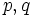# Finite solvable group

This article defines a property that can be evaluated for finite groups (and hence, a particular kind of group property)
View other properties of finite groups OR View all group properties

## Definition

A finite group is termed a finite solvable group if it satisfies the following equivalent conditions:

1. It is a solvable group
2. It is a polycyclic group
3. It has Sylow complements for all prime divisors of the order of the group
4. It has Hall subgroups of all possible orders
5. All its composition factors (i.e., the quotient groups for any composition series for the group) are cyclic groups of prime order. Equivalently, all its composition factors are abelian.
6. All its chief factors (i.e., the successive quotient groups for any chief series for the group) are elementary abelian groups.

### Equivalence of definitions

Further information: equivalence of definitions of finite solvable group

## Examples

### Examples based on order

We call a natural number$n$ a solvability-forcing number if every group of order$n$ is solvable. It turns out that:

• Any prime power is solvability-forcing, because prime power order implies nilpotent and nilpotent implies solvable.
• Any product of two prime powers, i.e., any number of the form$p^aq^b$, with$p,q$ primes, is solvability-forcing. See order has only two prime factors implies solvable (this result is also termed Burnside's$p^aq^b$-theorem).
• Any odd number is solvability-forcing. See odd-order implies solvable. This result, also called the odd-order theorem or the Feit-Thompson theorem, is highly nontrivial.
• Any square-free number i.e., any number that is a product

### Non-examples

Any finite simple non-abelian group is a finite group that is not solvable. See classification of finite simple groups for a list of finite simple non-abelian groups.

Further, any group that contains a finite simple non-abelian group as a subgroup, has a finite simple non-abelian group as a quotient group, or admits a finite simple non-abelian group as a subquotient must be non-solvable.

The smallest order examples of finite non-solvable groups are below:

## Relation with other properties

### Properties whose conjunction with finiteness gives this property

Below is a list of group properties such that a finite group has the property if and only if it is a finite solvable group.

Property Meaning Relation with solvability in general Intermediate properties between it and solvability in general
solvable group derived series reaches identity in finitely many steps same --
polycyclic group has a subnormal series (of finite length) with cyclic quotient groups stronger than solvability Finitely generated solvable group, Finitely presented solvable group|FULL LIST, MORE INFO
locally solvable group every finitely generated subgroup is solvable weaker than solvability |FULL LIST, MORE INFO
hypoabelian group the transfinite derived series reaches the identity element weaker than solvability Residually solvable group|FULL LIST, MORE INFO
residually solvable group every non-identity element is outside a normal subgroup for which the quotient group is solvable weaker than solvability |FULL LIST, MORE INFO

### Stronger properties

Property Meaning Proof of implication Proof of strictness (reverse implication failure) Intermediate notions
finite abelian group finite and an abelian group follows from abelian implies solvable see solvable not implies abelian Finite nilpotent group, Finite supersolvable group, Group having subgroups of all orders dividing the group order|FULL LIST, MORE INFO
finite nilpotent group finite and a nilpotent group follows from nilpotent implies solvable see solvable not implies nilpotent Finite supersolvable group, Group having a Sylow tower, Group having subgroups of all orders dividing the group order|FULL LIST, MORE INFO
finite supersolvable group finite and a supersolvable group follows from supersolvable implies solvable see solvable not implies supersolvable Group having subgroups of all orders dividing the group order|FULL LIST, MORE INFO